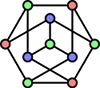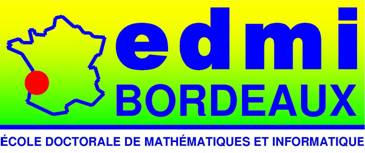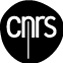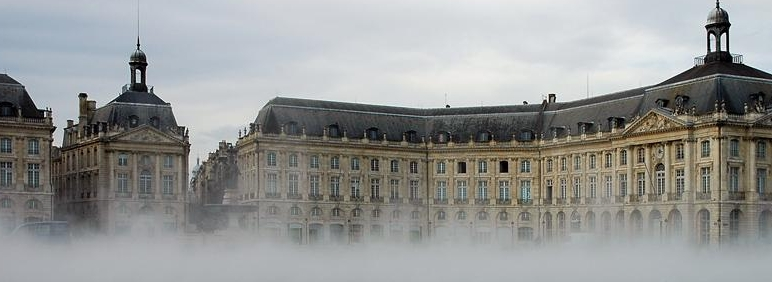Bordeaux Graph Workshop
Bordeaux, November 18-20, 2010.
LaBRI, Université Bordeaux 1, 351 cours de la libération, Talence, France.
 > An international workshop in honour of André Raspaud. Organized by the "Graphs and Applications" group.### Thursday, November 18, 2010

The workshop will start at 13:45.
• 13:45 - 14:00 Opening
• 14:00 - 15:00 Martin Skoviera, Local 3-edge-colourings of cubic graphs
• 15:00 - 16:00 Joergen Bang-Jensen, On the problem of finding disjoint cycles and dicycles in a digraph
• 16:00 - 16:30 Coffee break
• 16:30 - 17:30 Bjarne Toft, Reverse Hex - new proofs and new results
• 17:30 - 18:10 Daniel Gonçalves, SD-Delaunay Triangulations and the Duschnik-Miller Dimension of Simplicial Complexes
• 19:00 Wine and Cheese

### Friday, November 19, 2010

• 8:40 - 9:20 Ingo Schiermeyer, Progress on rainbow connection
• 9:20 - 10:20 Jean-Claude Bermond, Directed acyclic graphs with the unique dipath property
• 10:20 - 10:50 Coffee break
• 10:50 - 11:50 Xuding Zhu, Chromatic Ramsey number of graphs and fractional chromatic number of product graphs
• 11:50 - 12:30 Conference Picture
• 12:30 - 14:00 Lunch
• 14:00 - 15:00 Pavol Hell, List Homomorphisms
• 15:00 - 16:00 Alexandr Kostochka, Edge- and vertex-partitions of graphs with bounded maximum average degree
• 16:00 - 16:30 Coffee break
• 16:30 - 17:30 Gena Hahn, Vertex transitive cop-win graphs...and more
• 17:30 - 18:10 Guillaume Fertin, On some graph coloring problems
• 20:00 Banquet

### Saturday, November 20, 2010

• 9:00 - 10:00 Adrian Bondy, Induced Decompositions of Graphs
• 10:00 - 10:30 Coffee break
• 10:30 - 11:30 Jarik Nesetril, Orientations
• 11:30 - 12:10 Louis Esperet, Perfect matching in cubic graphs
The workshop will end at 12:10.

### Jorgen Bang-Jensen - On the problem of finding disjoint cycles and dicycles in a digraph

We consider the following problem: Given a digraph D, decide if there is a cycle B in D and a cycle C in its underlying undirected graph UG(D) such that V(B) ∩ V(C) = ∅. Whereas the problem is NP-complete if, as additional part of the input, a vertex x is prescribed to be contained in C, we give an overview of our recent proof that one can decide the existence of B,C in polynomial time under the (mild) additional assumption that D is strongly connected. Our methods actually find B,C in polynomial time if they exist. The behaviour of the problem as well as our solution depend on the cycle transversal number τ(D) of D, i.e. the smallest cardinality of a set T of vertices in D such that D-T is acyclic: If τ(D)≥3 then we employ McCuaig's framework on intercyclic digraphs to (always) find these cycles. If τ(D)=2 then we can characterize the digraphs for which the answer is "yes" by using topological methods relying on Thomassen's theorem on 2-linkages in acyclic digraphs. For the case τ(D)≤1 we provide an algorithm independent from any earlier work.

### Jean-Claude Bermond - Directed acyclic graphs with the unique dipath property

Let P be a family of dipaths of a DAG (Directed Acyclic Graph) G. The load of an arc is the number of dipaths containing this arc. Let π(G,P) be the maximum of the load of all the arcs and let w(G,P) be the minimum number of wavelengths (colors) needed to color the family of dipaths P in such a way that two dipaths with the same wavelength are arc-disjoint. There exist DAGs such that the ratio between w(G,P) and π(G,P) cannot be bounded. An internal cycle is an oriented cycle such that all the vertices have at least one predecessor and one successor in G (said otherwise every cycle contain neither a source nor a sink of G). We prove that, for any family of dipaths P, w(G, P) = π(G, P) if and only if G is without internal cycle. We also consider a new class of DAGs, which is of interest in itself, those for which there is at most one dipath from a vertex to another. We call these digraphs UPP-DAGs. For these UPP-DAGs we show that the load is equal to the maximum size of a clique of the conflict graph. We prove that the ratio between w(G,P) and π(G,P) cannot be bounded (a result conjectured in an other article). For that we introduce "good labelings" of the conflict graph associated to G and P, namely labelings of the edges such that for any ordered pair of vertices (x, y) there do not exist two paths from x to y with increasing labels.

### Adrian Bondy - Induced Decompositions of Graphs

We describe a new type of graph decomposition and present some basic results about it. This is joint work with Jayme Szwarcfiter.

### Louis Esperet - Perfect matching in cubic graphs

We prove that cubic bridgeless graph have exponentially many perfect matchings. This was conjectured in the mid-70's by Lovasz and Plummer. (joint work with F. Kardos, A. King, D. Kral, and S. Norin)

### Guillaume Fertin - On some Graph Coloring Problems

André Raspaud was the man who made me discover the delights of graph coloring. Since then, and though this is not my main line of research today, I sometimes like to play around with (and, if possible, contribute to) some graph coloring and graph labeling problems. In this talk, I will survey some results about graph coloring, with a focus on acyclic coloring. Time permits, I will also mention some results obtained with Stéphane Vialette (U. Paris-Est) on the so-called "S-labeling problem".

### Daniel Gonçalves - SD-Delaunay Triangulations and the Duschnik-Miller Dimension of Simplicial Complexes

In 1989, W. Schnyder gave a new charaterisation of planar graphs by means of the Duschnik-Miller dimension of their inclusion poset : A graph is planar if and only if its inclusion poset has Duschnik-Miller dimension at most 3. In 1999, P. Ossona de Mendez showed that simplicial complexes whose inclusion posets have Duschnik-Miller dimension bounded by d are linearly embeddable in Rd-1. Unfortunately the converse is only true for d ≤ 3. In this work we give a geometric characterization of simplicial complexes whose inclusion posets have Duschnik-Miller dimension d as special Delaunay triangulations of points in Rd-1. This generalizes a recent work of Bonichon et al for the case d=3.

### Gena Hahn - Vertex transitive cop-win graphs...and more

Many years ago, Nowakowski and Winkler, and, independently, Quilliot, characterized the graphs on which one cop can catch a single robber in the game where the cop begins and the two players alternately move along the edges of a reflexive graph. It is easy to see that there are no regular cop-win graphs that are not complete... if we demand that they be finite. In this talk we describe what we know for infinite graphs; in particular, we say why the finite-graph characterization does not work and we show that for every infinite cardinal there are as many non-isomorphic vertex-transitive cop-win graphs as possible. This is work with Bonato, Laviolette, Sauer, Tardif and Woodrow over the years.

### Pavol Hell - List homomorphisms and variants of interval graphs

I will describe a new combinatorial classification of the complexity of list homomorphisms. Previously, only an algebraic classification (due to A.A. Bulatov) was known for digraphs, although in the special case of graphs there existed more combinatorial classifications. The classification for graphs is closely related to the class of interval graphs, and these new generalizations suggest interesting new classes of digraphs, with many qualities resembling interval graphs.

### Alexandr Kostochka - Edge- and vertex-partitions of graphs with bounded maximum average degree

A convenient measure of sparseness of a graph G is the maximum average degree of G, mad(G):=max {2|E(H)|/|V(H)|: H ⊆ G}. For example, mad(G)<2g/(g-2) for every planar graph with girth g. Another measure of sparseness is the maximum degree, Δ(G), of G. Say that G is d-bounded, if Δ(G)≤d. We prove that every graph G with mad(G)<4-4/(d+2) decomposes into a forest and a d-bounded graph. The result is sharp, since for every d≥1, there are graphs G_d with mad(G_d)=4-4/(d+2) that do not have such decomposition. It implies several recent results on planar graphs with given girth. We also prove that every graph G with mad(G)≤12/5 contains an independent set I such that G-I is 1-bounded. This bound is sharp. Some results related to the two just described will be discussed. The talk is based on results joint with O. Borodin, S.-J. Kim, D. B. West, H. Wu, and X. Zhu.

### Jarik Nesetril - Orientations

The relationship between (undirected) graphs and their orientations is an example of perhaps the simplest expansion (lift) of finite structures. It leads to several interesting questions some of which will be surveyed in this talk. Particularly I want to concentrate on some topics related to our joint work with André.

### Ingo Schiermeyer - Progress on rainbow connection

An edge-coloured graph G is called rainbow connected if any two vertices are connected by a path whose edges have distinct colours. This concept of rainbow connection in graphs was introduced by Chartrand et al.. The rainbow connection number of a connected graph G, denoted rc(G), is the smallest number of colours that are needed in order to make G rainbow connected. In this talk we will present several lower and upper bounds for rc(G). We will discuss rc(G) for graphs with given minimum degree. Finally, we will present some recent Erdös-Gallai type results for rc(G).

### Martin Skoviera - Local 3-edge-colourings of cubic graphs

We develop the idea of a local 3-edge-colouring of a cubic graph, a generalisation of the usual 3-edge-colouring which uses an unlimited number of colours subject to the condition that, whenever two colours meet at a vertex, the third colour will always be the same. We describe local 3-edge-colourings in terms of colourings by points of a partial Steiner triple system such that the colours around each vertex form a block of the system. An important place in our investigation is held by colourings that use two smallest non-trivial Steiner triple systems, the Fano plane PG(2,2) and the affine plane AG(2,3). We also discuss the relationship of local 3-edge-colourings to several old and new conjectures concerning cubic graphs, including the Cycle Double Cover Conjecture, the Fulkerson conjecture, and the conjecture of Fan and Raspaud.

### Bjarne Toft - Reverse hex - New proofs and new results.

Reverse Hex, also known as Rex or Misčre Hex, is the variant of Hex in which the player who connects his/her two sides loses. After a brief survey of Reverse Hex, its history and all previous results on Reverse Hex, we update proofs and results for general nxn-boards. We give new simple proofs and strengthened versions of Lagarias and Sleator's theorem (each player can prolong the game until the board is full, so the first/second player can always win if n is even/odd), and of Evans'theorem (for even n, opening in the acute corner is a winning move). Also, for even n at least 4, we find another first-player winning opening (adjacent to the acute corner on the first player's side). Moreover, for n odd at least 3 and for each first-player opening, we find a second-player winning reply. Finally, in response to comments by Martin Gardener, we give simple winning strategies for board-size up to 5x5. This is joint work with Ryan Hayward, University of Alberta.

### Xuding Zhu - Chromatic Ramsey number of graphs and fractional chromatic number of product graphs

We write F→(G,G) if for any 2-edge colouring of F, there is a monochromatic copy of G. The chromatic Ramsey number Rχ(G) of G is defined as the minimum chromatic number of F for which F→(G,G). It is an easy observation that if χ(G)=n, then Rχ(G) ≥ (n-1)2+1. In 1976, Burr-Erdös-Lovasz conjectured that for any positive integer n, there exists an n-chromatic graph G with Rχ(G)=(n-1)2+1. This conjecture was verified for n ≤ 5 before. Recently, the author confirmed the whole conjecture. The proof of this conjecture involves an approach related to another well-known long standing conjecture: Hedetniemi's conjecture, which states that the categorical product of two n-chromatic graphs has chromatic number n. I proved the fractional version of this conjecture: For two graphs with fractional chromatic number at least r, their categorical product has fractional chromatic number at least r. It is by using this result that we settled Burr-Erdös-Lovasz conjecture.École doctorale Math-Info
GDR IM
ANR GRATOS
ANR GRATEL
ANR IDEA
CNRS
LaBRI
Maths ŕ Modeler
Université Bordeaux 1